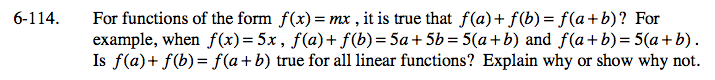### Home > A2C > Chapter 6 > Lesson 6.2.5 > Problem6-114

6-114.Think of a linear function that has a y-intercept other than 0 and use it to check the relationship f(a) + f(b) = f(a + b).

For example, try the linear function f(x) = 2x + 3 (you should think of a different one for your own answer).

f(a) + f(b) = (2a + 3) + (2b + 3)
f(a + b) = 2(a + b) + 3
f(a) + f(b) = 2a + 2b + 6

But, 2a + 2b + 6 ≠ 2a + 2b + 3

f(a) + f(b) = f(a + b) is not true for all linear functions.